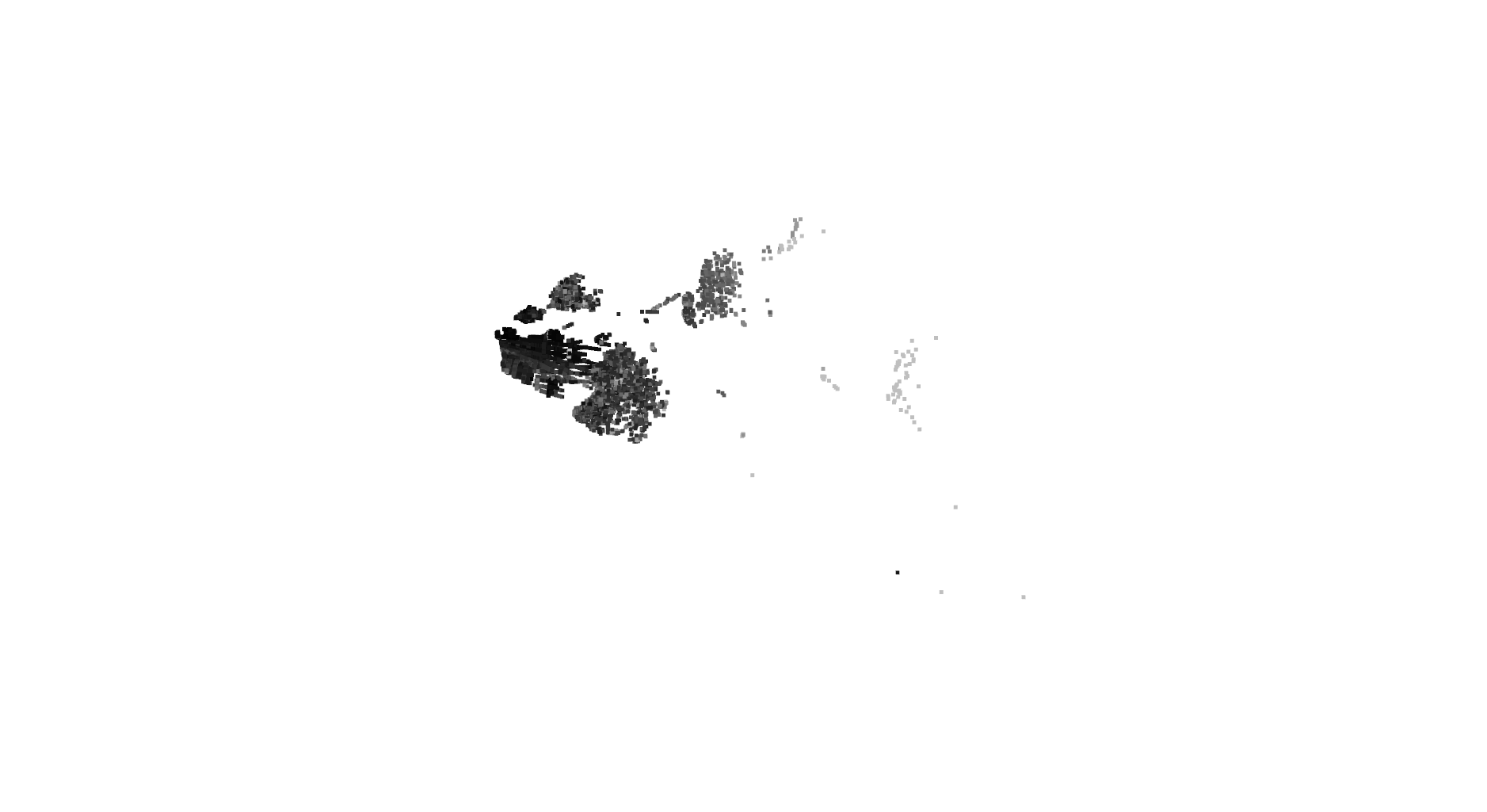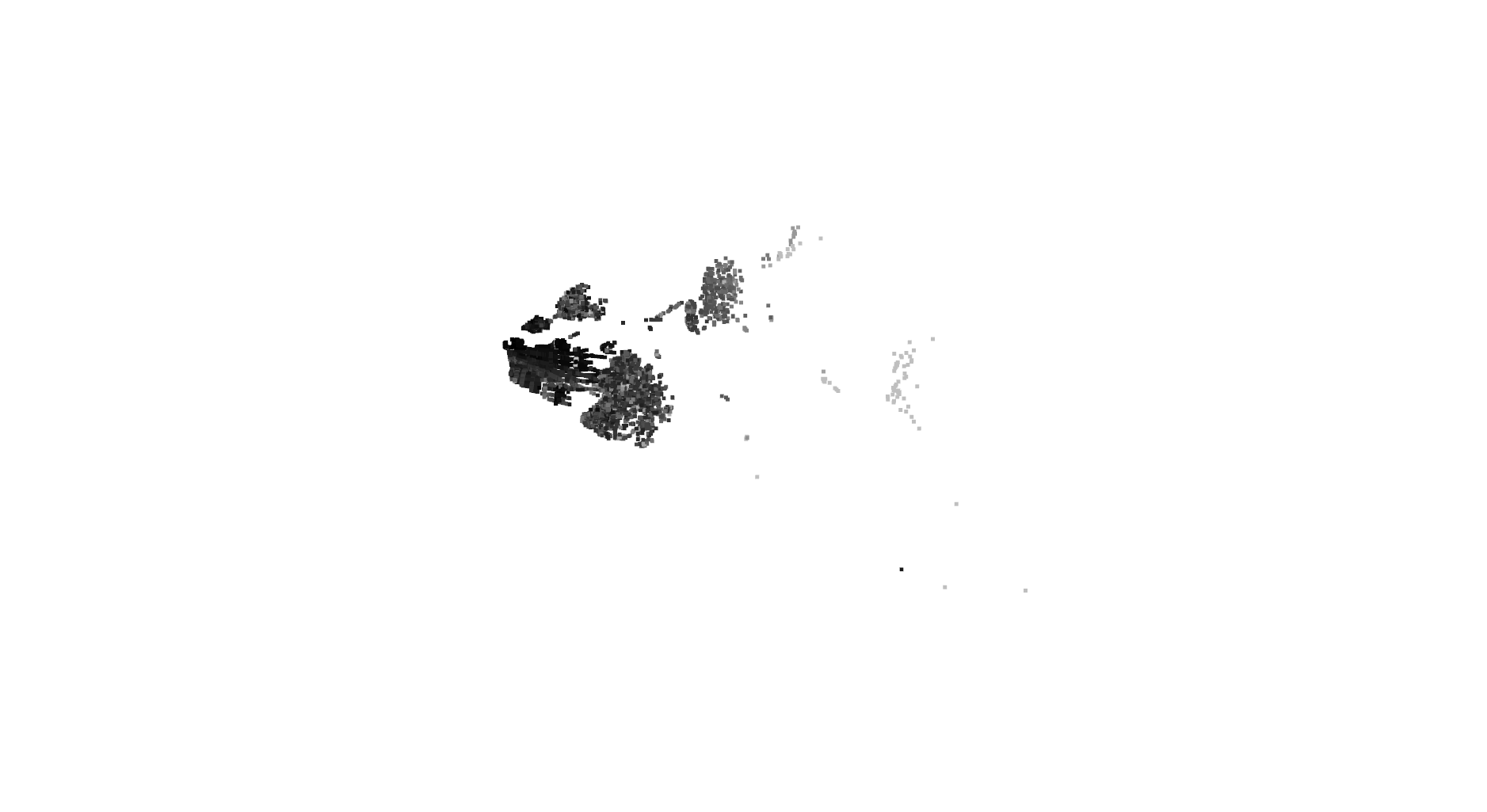Welcome to the tutorial on the A2D2 Dataset. In this tutorial, we will learn how to work with the configuration file, the view item, the LiDAR data, camera images, and 3D bounding boxes.

# Working with the configuration file¶

The configuration JSON file plays an important role in processing the A2D2 dataset.

In :
import json
import pprint


Open the configuration file

In :
with open ('cams_lidars.json', 'r') as f:


Show config file

In :
pprint.pprint(config)


The configuration file contains three main items:

In :
config.keys()


Each sensor in 'lidars', 'cameras', and 'vehicle' has an associated 'view'. A view is a sensor coordinate system, defined by an origin, an x-axis, and a y-axis. These are specified in cartesian coordinates (in m) relative to an external coordinate system. Unless otherwise stated the external coordinate system is the car's frame of reference.

'vehicle' contains a 'view' object specifying the frame of reference of the car. It also contains an 'ego-dimensions' object, which specifies the extension of the vehicle in the frame of reference of the car.

The 'lidars' object contains objects specifying the extrinsic calibration parameters for each LiDAR sensor. Our car has five LiDAR sensors: 'front_left', 'front_center', 'front_right', 'rear_right', and 'rear_left'. Each LiDAR has a 'view' defining its pose in the frame of reference of the car.

The 'cameras' object contains camera objects which specify their calibration parameters. The car has six cameras: 'front_left', 'front_center', 'front_right', 'side_right', 'rear_center' and 'side_left'. Each camera object contains:

• 'view'- pose of the camera relative to the external coordinate system (frame of reference of the car)
• 'Lens'- type of lens used. It can take two values: 'Fisheye' or 'Telecam'
• 'CamMatrix' - the intrinsic camera matrix of undistorted camera images
• 'CamMatrixOriginal' - the intrinsic camera matrix of original (distorted) camera images
• 'Distortion' - distortion parameters of original (distorted) camera images
• 'Resolution' - resolution (columns, rows) of camera images (same for original and undistorted images)
• 'tstamp_delay'- specifies a known delay in microseconds between actual camera frame times (default: 0)

Display the contents of 'vehicle':

In :
config['vehicle'].keys()


Likewise for LiDAR sensors:

In :
config['lidars'].keys()


Here we see the names of the LiDAR sensors mounted on the car. For example, the configuration parameters for the front_left LiDAR sensor can be accessed using

In :
config['lidars']['front_left']


The camera sensors mounted on the car can be obtained using

In :
config['cameras'].keys()


Configuration parameters for a particular camera can be accessed using e.g.

In :
config['cameras']['front_left']


## Working with view objects¶

We have seen that the vehicle and each sensor in the configuration file have a 'view' object. A view specifies the pose of a sensor relative to an external coordinate system, here the frame of reference of the car. In the following we use the term 'global' interchangeably with 'frame of reference of the car'.

A view associated with a sensor can be accessed as follows:

In :
view = config['cameras']['front_left']['view']

In :
import numpy as np
import numpy.linalg as la


Define a small constant to avoid errors due to small vectors.

In :
EPSILON = 1.0e-10 # norm should not be small


The following functions get the axes and origin of a view.

In :
def get_axes_of_a_view(view):
x_axis = view['x-axis']
y_axis = view['y-axis']

x_axis_norm = la.norm(x_axis)
y_axis_norm = la.norm(y_axis)

if (x_axis_norm < EPSILON or y_axis_norm < EPSILON):
raise ValueError("Norm of input vector(s) too small.")

# normalize the axes
x_axis = x_axis / x_axis_norm
y_axis = y_axis / y_axis_norm

# make a new y-axis which lies in the original x-y plane, but is orthogonal to x-axis
y_axis = y_axis - x_axis * np.dot(y_axis, x_axis)

# create orthogonal z-axis
z_axis = np.cross(x_axis, y_axis)

# calculate and check y-axis and z-axis norms
y_axis_norm = la.norm(y_axis)
z_axis_norm = la.norm(z_axis)

if (y_axis_norm < EPSILON) or (z_axis_norm < EPSILON):
raise ValueError("Norm of view axis vector(s) too small.")

# make x/y/z-axes orthonormal
y_axis = y_axis / y_axis_norm
z_axis = z_axis / z_axis_norm

return x_axis, y_axis, z_axis

In :
def get_origin_of_a_view(view):
return view['origin']


A homogeneous transformation matrix from view point to global coordinates (inverse "extrinsic" matrix) can be obtained as follows. Note that this matrix contains the axes and the origin in its columns.

In :
def get_transform_to_global(view):
# get axes
x_axis, y_axis, z_axis = get_axes_of_a_view(view)

# get origin
origin = get_origin_of_a_view(view)
transform_to_global = np.eye(4)

# rotation
transform_to_global[0:3, 0] = x_axis
transform_to_global[0:3, 1] = y_axis
transform_to_global[0:3, 2] = z_axis

# origin
transform_to_global[0:3, 3] = origin

return transform_to_global


For the view defined above

In :
transform_to_global = get_transform_to_global(view)
print (transform_to_global)

[[ 9.96714314e-01 -8.09890350e-02  1.16333982e-03  1.71104606e+00]
[ 8.09967396e-02  9.96661051e-01 -1.03090934e-02  5.80000039e-01]
[-3.24531964e-04  1.03694477e-02  9.99946183e-01  9.43144935e-01]
[ 0.00000000e+00  0.00000000e+00  0.00000000e+00  1.00000000e+00]]


Homogeneous transformation matrix from global coordinates to view point coordinates ("extrinsic" matrix)

In :
def get_transform_from_global(view):
# get transform to global
transform_to_global = get_transform_to_global(view)
trans = np.eye(4)
rot = np.transpose(transform_to_global[0:3, 0:3])
trans[0:3, 0:3] = rot
trans[0:3, 3] = np.dot(rot, -transform_to_global[0:3, 3])

return trans


For the view defined above

In :
transform_from_global = get_transform_from_global(view)
print(transform_from_global)

[[ 9.96714314e-01  8.09967396e-02 -3.24531964e-04 -1.75209613e+00]
[-8.09890350e-02  9.96661051e-01  1.03694477e-02 -4.49267371e-01]
[ 1.16333982e-03 -1.03090934e-02  9.99946183e-01 -9.39105431e-01]
[ 0.00000000e+00  0.00000000e+00  0.00000000e+00  1.00000000e+00]]


The transform_to_global and transform_from_global matrices should be the inverse of one another. Check that muliplying them results in an identity matrix (subject to numerical precision):

In :
print(np.matmul(transform_from_global, transform_to_global))

[[ 1.00000000e+00 -4.05809291e-18 -7.51833703e-21  0.00000000e+00]
[-4.05809291e-18  1.00000000e+00 -5.22683251e-19  5.55111512e-17]
[-7.51833703e-21 -5.22683251e-19  1.00000000e+00  0.00000000e+00]
[ 0.00000000e+00  0.00000000e+00  0.00000000e+00  1.00000000e+00]]


The global-to-view rotation matrix can be obtained using

In :
def get_rot_from_global(view):
# get transform to global
transform_to_global = get_transform_to_global(view)
# get rotation
rot =  np.transpose(transform_to_global[0:3, 0:3])

return rot


For the view defined above

In :
rot_from_global = get_rot_from_global(view)
print(rot_from_global)

[[ 9.96714314e-01  8.09967396e-02 -3.24531964e-04]
[-8.09890350e-02  9.96661051e-01  1.03694477e-02]
[ 1.16333982e-03 -1.03090934e-02  9.99946183e-01]]


The rotation matrix from this view point to the global coordinate system can be obtained using

In :
def get_rot_to_global(view):
# get transform to global
transform_to_global = get_transform_to_global(view)
# get rotation
rot = transform_to_global[0:3, 0:3]

return rot


For the view defined above

In :
rot_to_global = get_rot_to_global(view)
print(rot_to_global)

[[ 9.96714314e-01 -8.09890350e-02  1.16333982e-03]
[ 8.09967396e-02  9.96661051e-01 -1.03090934e-02]
[-3.24531964e-04  1.03694477e-02  9.99946183e-01]]


Let us see how we can calculate a rotation matrix from a source view to a target view

In :
def rot_from_to(src, target):
rot = np.dot(get_rot_from_global(target), get_rot_to_global(src))

return rot


A rotation matrix from front left camera to front right camera

In :
src_view = config['cameras']['front_left']['view']
target_view = config['cameras']['front_right']['view']
rot = rot_from_to(src_view, target_view)
print(rot)

[[ 0.99614958 -0.0876356  -0.00245312]
[ 0.08757611  0.99598808 -0.01838914]
[ 0.00405482  0.01810349  0.9998279 ]]


A rotation matrix in the opposite direction (front right camera -> front left camera)

In :
rot = rot_from_to(target_view, src_view)
print(rot)

[[ 0.99614958  0.08757611  0.00405482]
[-0.0876356   0.99598808  0.01810349]
[-0.00245312 -0.01838914  0.9998279 ]]


In the same manner, we can also calculate a transformation matrix from a source view to a target view. This will give us a 4x4 homogeneous transformation matrix describing the total transformation (rotation and shift) from the source view coordinate system into the target view coordinate system.

In :
def transform_from_to(src, target):
transform = np.dot(get_transform_from_global(target), \
get_transform_to_global(src))

return transform


A transformation matrix from front left camera to front right camera

In :
src_view = config['cameras']['front_left']['view']
target_view = config['cameras']['front_right']['view']
trans = transform_from_to(src_view, target_view)
print(trans)

[[ 0.99614958 -0.0876356  -0.00245312 -0.00769387]
[ 0.08757611  0.99598808 -0.01838914  1.1599368 ]
[ 0.00405482  0.01810349  0.9998279   0.00935439]
[ 0.          0.          0.          1.        ]]


A transformation matrix in the opposite direction (front right camera -> front left camera)

In :
transt = transform_from_to(target_view, src_view)
print (transt)

[[ 0.99614958  0.08757611  0.00405482 -0.09395644]
[-0.0876356   0.99598808  0.01810349 -1.15612684]
[-0.00245312 -0.01838914  0.9998279   0.01195858]
[ 0.          0.          0.          1.        ]]


Check if the product of the two opposite transformations results in a near identity matrix.

In :
print(np.matmul(trans, transt))

[[ 1.00000000e+00  9.38784819e-18 -2.59997757e-19 -4.15466272e-16]
[ 9.38784819e-18  1.00000000e+00 -1.78819878e-18  0.00000000e+00]
[-2.59997757e-19 -1.78819878e-18  1.00000000e+00 -5.20417043e-18]
[ 0.00000000e+00  0.00000000e+00  0.00000000e+00  1.00000000e+00]]


We have seen that by using views we can transform coordinates from one sensor to another, or from a sensor to global global coordinates (and vice versa). In the following section, we read point clouds corresponding to all cameras. The point clouds are in camera view coordinates. In order to get a coherent view of the point clouds, we need to transform them into global coordinates.

# Working with LiDAR data¶

First, read a LiDAR point cloud corresponding to the front center camera. The LiDAR data is saved in compressed numpy format, which can be read as follows:

In :
from os.path import join
import glob

root_path = './camera_lidar_semantic_bboxes/'
# get the list of files in lidar directory
file_names = sorted(glob.glob(join(root_path, '*/lidar/cam_front_center/*.npz')))

# select the lidar point cloud
file_name_lidar = file_names



Let us explore the LiDAR data using the LiDAR points within the field of view of the front center camera. List keys:

In :
print(list(lidar_front_center.keys()))

['azimuth', 'row', 'lidar_id', 'depth', 'reflectance', 'col', 'points', 'timestamp', 'distance']


Get 3D point measurements

In :
points = lidar_front_center['points']


Get reflectance measurements

In :
reflectance = lidar_front_center['reflectance']


Get timestamps

In :
timestamps = lidar_front_center['timestamp']


Get coordinates of LiDAR points in image space

In :
rows = lidar_front_center['row']
cols = lidar_front_center['col']


Get distance and depth values

In :
distance = lidar_front_center['distance']
depth = lidar_front_center['depth']


Since the car is equipped with five LiDAR sensors, you can get the LiDAR sensor ID of each point using

In :
lidar_ids = lidar_front_center['lidar_id']


One way of visualizing point clouds is to use the Open3D library. The library supports beyond visualization other functionalities useful for point cloud processing. For more information on the library please refer to http://www.open3d.org/docs/release/.

In :
import open3d as o3


To visualize the LiDAR point clouds, we need to create an Open3D point cloud from the 3D points and reflectance values. The following function generates colors based on the reflectance values.

In :
# Create array of RGB colour values from the given array of reflectance values
def colours_from_reflectances(reflectances):
return np.stack([reflectances, reflectances, reflectances], axis=1)



Now we can create Open3D point clouds for visualization

In :
def create_open3d_pc(lidar, cam_image=None):
# create open3d point cloud
pcd = o3.geometry.PointCloud()

# assign point coordinates
pcd.points = o3.utility.Vector3dVector(lidar['points'])

# assign colours
if cam_image is None:
median_reflectance = np.median(lidar['reflectance'])
colours = colours_from_reflectances(lidar['reflectance']) / (median_reflectance * 5)

# clip colours for visualisation on a white background
colours = np.clip(colours, 0, 0.75)
else:
rows = (lidar['row'] + 0.5).astype(np.int)
cols = (lidar['col'] + 0.5).astype(np.int)
colours = cam_image[rows, cols, :] / 255.0

pcd.colors = o3.utility.Vector3dVector(colours)

return pcd


Generate Open3D point cloud for the LiDAR data associated with the front center camera

In :
pcd_front_center = create_open3d_pc(lidar_front_center)


Visualize the point cloud

In :
o3.visualization.draw_geometries([pcd_front_center])Let us transform LiDAR points from the camera view to the global view.

First, read the view for the front center camera from the configuration file:

In :
src_view_front_center = config['cameras']['front_center']['view']


The vehicle view is the global view

In :
vehicle_view = target_view = config['vehicle']['view']


The following function maps LiDAR data from one view to another. Note the use of the function 'transform_from_to'. LiDAR data is provided in a camera reference frame.

In :
def project_lidar_from_to(lidar, src_view, target_view):
lidar = dict(lidar)
trans = transform_from_to(src_view, target_view)
points = lidar['points']
points_hom = np.ones((points.shape, 4))
points_hom[:, 0:3] = points
points_trans = (np.dot(trans, points_hom.T)).T
lidar['points'] = points_trans[:,0:3]

return lidar


Now project the LiDAR points to the global frame (the vehicle frame of reference)

In :
lidar_front_center = project_lidar_from_to(lidar_front_center,\
src_view_front_center, \
vehicle_view)


Create open3d point cloud for visualizing the transformed points

In :
pcd_front_center = create_open3d_pc(lidar_front_center)


Visualise:

In :
o3.visualization.draw_geometries([pcd_front_center])# Working with images¶

Import the necessary packages for reading, saving and showing images.

In :
import cv2
%matplotlib inline
import matplotlib.pylab as pt


Let us load the image corresponding to the above point cloud.

In :
def extract_image_file_name_from_lidar_file_name(file_name_lidar):
file_name_image = file_name_lidar.split('/')
file_name_image = file_name_image[-1].split('.')
file_name_image = file_name_image.split('_')
file_name_image = file_name_image + '_' + \
'camera_' + \
file_name_image + '_' + \
file_name_image + '.png'

return file_name_image

In :
seq_name = file_name_lidar.split('/')
file_name_image = extract_image_file_name_from_lidar_file_name(file_name_lidar)
file_name_image = join(root_path, seq_name, 'camera/cam_front_center/', file_name_image)


Display image

In :
image_front_center = cv2.cvtColor(image_front_center, cv2.COLOR_BGR2RGB)

In :
pt.fig = pt.figure(figsize=(15, 15))

# display image from front center camera
pt.imshow(image_front_center)
pt.axis('off')
pt.title('front center')

Out:
Text(0.5, 1.0, 'front center')